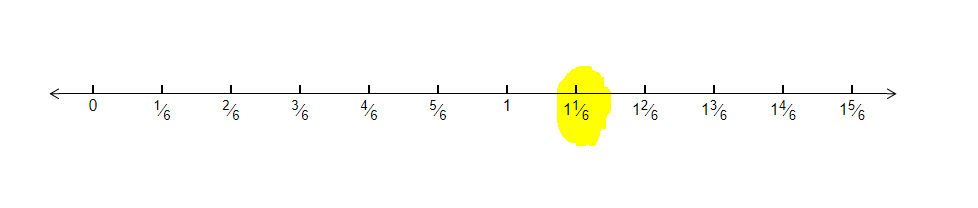# How can we represent $\frac{7}{6}$ on the number line?

Given: The number give to us is $\frac{7}{6}$

To do:  Represent it on number line

Solution:

Let us represent $\frac{7}{6}$ on number line.

First Draw a number line and mark 0, 1 and 2.

In $\frac{7}{6}$, the denominator is 6, so we divide the line between 0 and 1 into 6 equal parts. Similarly we divide between '1' and '2' into 6 equal parts.

Since numerator is 7, the seventh marking after 0 will give $\frac{7}{6}$.Updated on: 10-Oct-2022

235 Views